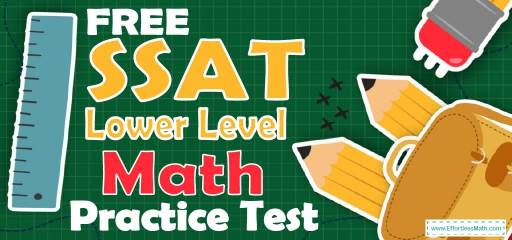# FREE SSAT Lower Level Math Practice TestWelcome to our FREE SSAT Lower Level Math practice test, with answer key and answer explanations. This practice test’s realistic format and high-quality practice questions can help you succeed on the SSAT Lower Level Math test. Not only does the test closely match what you will see on the real SSAT Lower Level, but it also comes with detailed answer explanations.

For this practice test, we’ve selected 20 real questions from past exams for your SSAT Lower Level Practice test. You will have the chance to try out the most common SSAT Lower Level Math questions. For every question, there is an in-depth explanation of how to solve the question and how to avoid mistakes next time.

Use our free SSAT Lower Level Math practice tests and study resources (updated for 2022) to ace the SSAT Lower Level Math test! Make sure to follow some of the related links at the bottom of this post to get a better idea of what kind of mathematics questions you need to practice.

## 10 Sample SSAT Lower Level Math Practice Questions

1- A square has an area of $$64 \space cm^2$$. What is its perimeter?

A. 30

B. 32

C. 34

D. 36

2- Find the missing number in the sequence: $$(5, 8, 12, ….., 23)$$

A. 15

B. 17

C. 18

D. 20

E. 22

3- The length of a rectangle is 3 times its width. If the length is 18, what is the perimeter of the rectangle?

A. 24

B. 30

C. 36

D. 45

E. 56

4- Mary has $$y$$ dollars. John has $10 more than Mary. If John gives Mary$12, then in terms of $$y$$, how much does John have now?

A. $$y+1$$

B. $$y+10$$

C. $$y−2$$

D. $$y−1$$

E. $$y+3$$

5- Dividing 107 by 6 leaves a remainder of?

A. 1

B. 2

C. 3

D. 4

E. 5

6- If $$6,000+A−200=7,400$$, then $$A =$$

A. 200

B. 600

C. 1600

D. 2200

E. 3000

7- A square has an area of $$49 \space cm^2$$. What is the perimeter of the square?

A. 14 cm

B. 28 cm

C. 35 cm

D. 49 cm

E.63 cm

8- Which of the following fractions is less than $$\frac{3}{2}$$?

A. 1.4

B. $$\frac{5}{2}$$

C. 3

D. 2.8

E.3.2

9- Use the equation below to answer the question.
$$x+3=6$$ $$2y=8$$
What is the value of $$y−x$$?

A. 1

B. 2

C. 3

D. 4

E. 5

10- If $$310−x+116=225$$, then $$x=$$

A. 101

B. 156

C. 201

D. 211

E. 310

11- If $$x∎y=3x+y−2$$, what is the value of $$4∎12$$?

A. 4

B. 18

C. 22

D. 36

E.48

12- Of the following, which number is the greatest?

A. 0.092

B. 0.8913

C. 0.8923

D. 0.8896

E. 0.88

13- Solve: $$\frac{7}{8}−\frac{3}{4}=$$?

A. 0.125

B. 0.375

C. 0.5

D. 0.625

E. 0.775

14- Which of the following is the closest to $$4.02$$?

A. 4

B. 4.2

C. 4.3

D. 4.5

E. 3.5

15- Which of the following statements is False?

A. $$(7×2+14)×2=56$$

B. $$(2×5+4)÷2=7$$

C. $$3+(3×6)=21$$

D. $$4×(3+9)=48$$

E.$$14÷(2+5)=5$$

16- When 78 is divided by 5, the remainder is the same as when 45 is divided by

A. 2

B. 4

C. 5

D. 7

D. 9

17- John has 2,400 cards and Max has 606 cards. How many more cards does John have than Max?

A. 1,794

B. 1,798

C. 1,812

D. 1,828

E. 1,994

18- In the following right triangle, what is the value of $$x$$?

A. 15

B. 30

C. 45

D. 60

E. It cannot be determined from the information given

19- What is 20 percent of 120?

A. 20

B. 24

C. 30

D. 40

E.44

20- In a basket, the ratio of red marbles to blue marbles is 3 to 2. Which of the following could NOT be the total number of red and blue marbles in the basket?

A. 15

B. 32

C. 55

D. 60

E. 70

## Best SSAT Lower Level Math Prep Resource for 2022

1- B
Area of a square = $$side×side=64→side=8$$
Perimeter of a square = $$4 × side = 4×8=32$$

2- B
$$5+3=8 ,8+4=12$$
$$12+5=17$$
$$17+6=23$$

3- D
The length of the rectangle is 18. Then, its width is 6.
$$18 ÷ 3 = 6$$
Perimeter of a rectangle$$2×width+2×length=2×6+2×18=12+36=48$$

4- C
Mary’ s Mone$$y=y$$
John’ s Money$$=y+10$$
John gives Mary $$12→y+10-12=y-2$$

5- E
Dividing 107 by 6 leaves a remainder of 5.

6- C
$$6,000+A-200=7,400→6,000+A=7,400+200=7,600→A=7,600-6,000=1,600$$

7- B
Area of a square = $$side ×side=49→side=7$$
Perimeter of a square=$$4×side=4×7=28$$

8- A
$$\frac{3}{2}=1.5>1.4$$

9- A
$$x+3=6→x=3$$
$$2y=8→y=4$$
$$y-x=4-3=1$$

10- C
$$310-x+116=225→310-x=225-116=109→x=310-109=201$$

11- C
If $$x∎y=3x+y-2$$, Then:
$$4∎12=3(4)+12-2=12+12-2=22$$

12- C
Of the numbers provided, $$0.8923$$ is the greatest.

13- A
$$\frac{7}{8}-\frac{3}{4}=\frac{7}{8}-\frac{6}{8}=\frac{1}{8}=0.125$$

14- A
The closest number to 4.02 is 4.

15- E
$$14÷(2+5)=14÷7=2$$

16- C
78 divided by 5, the remainder is 3. 45 divided by 7, the remainder is also 3.

17- A
$$2400-606=1794$$

18- C
All angles in a triangle sum up to 180 degrees. The triangle provided is isosceles. In an isosceles triangle, the three angles are 45, 45, and 90 degrees. Therefore, the value of $$x$$ is 45.

19- B
20 percent of $$120=\frac{20}{100}×120=\frac{1}{5}×120=24$$

20- B
The ratio of red marbles to blue marbles is 3 to 2. Therefore, the total number of marbles must be divisible by 5.
$$3 + 2 = 5$$
32 is the only one that is not divisible by 5.

Looking for the best resource to help you succeed on the SSAT Lower Level Math test?

## The Best Books to Ace the SSAT Lower Level Math Test

### What people say about "FREE SSAT Lower Level Math Practice Test - Effortless Math: We Help Students Learn to LOVE Mathematics"?

No one replied yet.

X
30% OFF

Limited time only!

Save Over 30%

SAVE $5 It was$16.99 now it is \$11.99